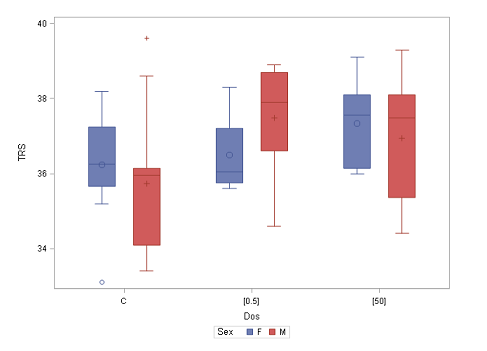7
Oct

31
Oct
Uppsala

# Statistics I: Basic statistics, 4 hpThe Ph.D. course will be given in Uppsala 7 - 31 October 2019.

Prerequisites: None

Objective:
The objective of the course is to give an introduction to basic probability theory and statistical inference. On completion of the course, the student will be able to:

• apply basic probability concepts and statistical principles and formulate a problem in statistical terms
• describe common statistical methods including conditions and assumptions
• select an appropriate statistical method for a given problem
• carry out a basic statistical analysis in the statistical software R or SAS
• interpret and evaluate results correctly and draw reasonable conclusions
• clearly and concisely communicate results and conclusions

Content
The course is built on lectures and computer exercises. The main components are as follows:

• Descriptive statistics.
• Basic probability theory.
• Probability distributions.
• Statistical inference of means and proportions, one and two samples.
• Nonparametric methods.
• Analysis of frequency data.
Facts
Time: 2019-10-07 - 2019-10-31
City: Uppsala
Last signup date: 11 September 2019

Applications are to be sent by e-mail to Claudia.von.Bromssen@slu.se and should contain the following information:

Name of applicant • Personal identification number (personnummer) • Academic affiliation.

Related pages:

ContactPage editor: hilda.edlund@slu.se